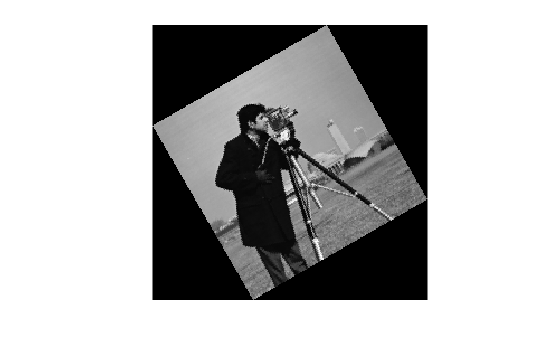# Find Image Rotation and Scale

This example shows how to align or register two images that differ by a rotation and a scale change. You can calculate the rotation angle and scale factor and transform the distorted image to recover the original image.

Read an image into the workspace.

```original = imread("cameraman.tif"); imshow(original) text(size(original,2),size(original,1)+15, ... "Image courtesy of Massachusetts Institute of Technology", ... FontSize=7,HorizontalAlignment="right")```### Step 2: Resize and Rotate the Image

Create a distorted version of the image by resizing and rotating the image. Note that `imrotate` rotates images in a counterclockwise direction when you specify a positive angle of rotation.

```scaleFactor = 0.7; distorted = imresize(original,scaleFactor); theta = 30; distorted = imrotate(distorted,theta); imshow(distorted)```### Step 3: Select Control Points

This example specifies three pairs of control points.

```movingPoints = [128.6 75.4; 151.9 163.9; 192.1 118.6]; fixedPoints = [169.1 73.6; 135.6 199.9; 217.1 171.9];```

If you want to pick the control points yourself, then you can use the Control Point Selection Tool. Open this tool by using the `cpselect` function.

```[movingPoints,fixedPoints] = cpselect(distorted,original,"Wait",true); ```

### Step 4: Estimate Affine Transformation

Fit a geometric transformation to your control points using the `fitgeotform2d` function. This example fits a similarity transformation because the distortion consists only of rotation and isotropic scaling.

`tform = fitgeotform2d(movingPoints,fixedPoints,"similarity");`

### Step 5: Recover Scale Factor and Rotation Angle

The geometric transformation, `tform`, represents how to transform the moving image to the fixed image. If you want to determine the scale factor and rotation angle that you applied to the fixed image to create the moving image, then use the inverse of the geometric transformation.

`tformInv = invert(tform)`
```tformInv = simtform2d with properties: Dimensionality: 2 Scale: 0.7014 RotationAngle: -29.6202 Translation: [0.0051 89.0695] R: [2x2 double] A: [3x3 double] ```

The values of the `Scale` property should match the value of `scaleFactor` that you set in Step 2: Resize and Rotate the Image.

The value of the `RotationAngle` property should have the same magnitude as the angle `theta` that you set in Step 2: Resize and Rotate the Image. However, the angle in `RotationAngle` has the opposite sign as `theta`. The sign is opposite because the `simtform2d` object stores the rotation angle as the amount of rotation from the positive x-axis to the positive y-axis in intrinsic coordinates. For images, the positive x direction points to the right and the positive y axis points downward, therefore a positive rotation angle is in the clockwise direction. A positive rotation angle in the clockwise direction corresponds to a negative rotation angle in the counterclockwise direction, and vice versa.

### Step 6: Recover Original Image

Recover the original image by transforming `distorted`, the rotated-and-scaled image, using the geometric transformation `tform` and what you know about the spatial referencing of `original`. Use the `OutputView` name-value argument to specify the resolution and grid size of the resampled output image.

```Roriginal = imref2d(size(original)); recovered = imwarp(distorted,tform,OutputView=Roriginal);```

Compare `recovered` to `original` by looking at them side-by-side in a montage.

`montage({original,recovered})`The recovered (right) image quality does not match the original (left) image because of the distortion and recovery process. In particular, the image shrinking causes information loss. The artifacts around the edges are due to the limited accuracy of the transformation. If you were to pick more points in Step 3: Select Control Points, the transformation would be more accurate.